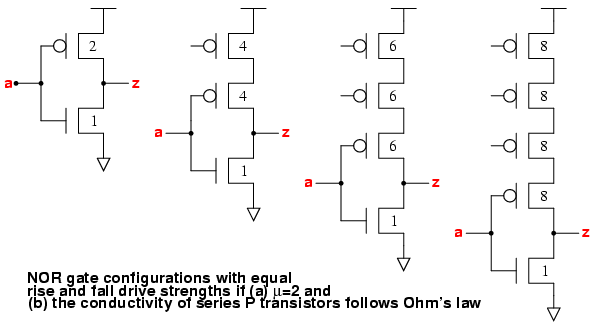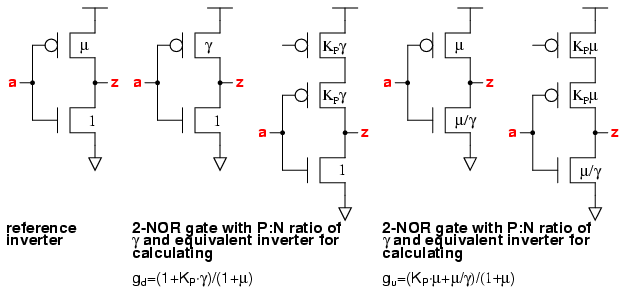## Logical Effort of NOR Gates

UP PREV NEXTThe calculation of the logical effort of NOR gates follows a parallel path to the one used for NAND gates. We size the series P transistors so that their conductance is the same as the single transistors in a reference inverter. We define the amount by which the transistor must be increased as KP, the P transistor conductivity coefficient.

In the book Logical Effort, Ohm's law is used so that KP is 2 for a 2-NOR gate, 3 for a 3-NOR gate and 4 for a 4-NOR gate. The book recognises that this is a simplification, because velocity saturation of the carriers means that series combinations of P transistors are more conductive than a single one. However, the lower speed of holes compared to electrons means that the speed of the holes in a single P transistor is not so subject to velocity saturation effects, so that KP following Ohm's law is a more accurate approximation than it was for NAND gates.

Following the Logical Effort book example, we apply Ohm's law and set µ=2. We can see that the ratio of the input capacitance of 2-, 3- and 4-input NOR gates compared to a reference inverter is (4+1)/(2+1), (6+1)/(2+1) and (8+1)/(2+1).

 gate INV 2-NOR 3-NOR 4-NOR input cap 3 5 7 9 logical effort g 1 5/3 7/3 9/3 1.00 1.67 2.33 3.00

### Selecting the P:N Transistor Ratio for 2/3/4 Input NOR GatesHere we consider a more general case where the NOR gate can have a P:N transistor ratio of γ; the N:P conductivity = µ; and the value for KP of the NOR gates need not be equal to the number of series P transistors. A 2-input NOR gate with the equivalent drive of an inverter with (P=γ,N=1) has (P=KP·γ,N=1). This matches the conductivity of the N transistor of the reference inverter, so that the falling logical effort
gd = (1+KP·γ)/(1+µ)
For the rising logical effort, we scale the P transistor of the equivalent inverter to P=µ. The series P transistor of the NOR gate is then P=KP·µ,so that
gu = (KP·µ+µ/γ)/(1+µ)
The logical effort g is then:
g = ½×(KP·γ+1+KP·µ+µ/γ)/(1+µ)

For the vsclib, the values used are µ=2.25 and KN=(15/8, 22/8, 29/8) for 2-,3-,4-input NOR gates. These values for KP are empirical, and come from an analysis of the logical effort of NOR gates from various standard cell libraries.

The fastest NOR gate occurs when
dg/dγ = 0 = KP-µ2
from which γ = √(µ/KP) for the fastest NOR gates.

Some values of logical effort for 2-NOR gates with different values of γ, assuming that µ=2.25, and KP=15/8 are:

 P:N ratio γ 0.5 1 1.1 1.5 2 2.25 2.5 3 logical effort g 1.64 1.44 1.43 1.47 1.55 1.61 1.66 1.78

The minimum logical effort occurs when γ=√(2.25×8/15)=1.10. For NOR gates, the problem is that at the values of γ which give the fastest speed, the skew between the rise and fall drive strengths is very large. Furthermore, for noise considerations we don't want γ to be smaller than 1. So for the vsclib, we choose for 2-NOR gates γ=2 to match inverters, but will also include a family of 2-NOR gates with γ=1.1 for the fastest speed.

The table below shows some values of logical effort for 3-NOR gates with different values of γ, assuming that µ=2.25, and KP=22/8.

 P:N ratio γ 0.5 0.9 1 1.5 2 2.25 2.5 3 logical effort g 2.01 1.87 1.88 1.97 2.13 2.21 2.3 2.49

The minimum logical effort occurs when γ=√(2.25×8/22)=0.905. Here again we set γ=2 for regular 3-NOR gates, and provide a family of gates with γ=1. We can't provide the fastest 3-NOR gates because these have γ<1, which we don't allow because of noise concerns.

The table below shows some values of logical effort for 4-NOR gates with different values of γ, assuming that µ=2.25, and KP=29/8.

 P:N ratio γ 0.5 0.79 1 1.5 2 2.25 2.5 3 logical effort g 2.38 2.29 2.31 2.48 2.7 2.82 2.94 3.2

The minimum logical effort occurs when γ=√(2.25×8/29)=0.788. As with the 3-NOR, also for the 4-NOR gates, γ is set to 2 with an extra family of 4-NOR gates having γ=1.

The vsclib choice for NOR gates matches that in Logical Effort. The P:N transistor ratio γ is chosen to be 2 in order to keep a reasonable balance between the rise and fall drive strengths. The P transistor conductivity coefficient is slightly different, leading to a difference in the logical efforts. These are compared with the average values of NOR gate logical effort in Table 5.4 of the book.

 Gate 2-NOR 3-NOR 4-NOR Logical Effort theoretical g 1.67 2.33 3.00 Theoretical g for vsclib 1.55 2.13 2.70 Average g of 9 processes 1.54 2.08 2.63

From this we can conclude that the choices made for P transistor conductivity coefficients, KP for the vsclib NAND gates are OK. This is important, since the values of KP used to derive the theoretical logical effort values in the vsclib are empirical. If they don't lead to an improvement over a simple application of Ohm's law, then the analysis has not been well done!